Coming to Statistica
in 2022/2023

Group Classes

Interactive Webinars

Blog

Guestbook

.

E-mail        anne@statistica.com.au

# Place         Willetton, Western Australia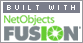# Mathematics & Statistic Tutor Perth - SPSS HelpChi-Square Test of Independence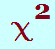Tests of Significance   Normal distribution
SPSS  Instructions

(a)  Write down the linear regression equation.

(b) What is the value of the standard error of the estimate?

(c) How many degrees of freedom are associated with the t-value for the line of regression?

(d) What is the value of the correlation coefficient?

(e)  Confidence and Prediction Interval

(f) What is the 95% confidence interval for the mean value of   Ŷ when x =   ?

(g) What is the 95% prediction interval for    Ŷ   when x =   ?

What I have tried to do here is put as simply as possible how to answer a variety of questions using SPSS output. The following tables are an example of the output and then I have shown where the information is to answer certain questions.

Coefficients

 Model UnstandardisedCoefficients Standardised  Coefficients t Sig B Std  Error Beta Constant Additive 2.129  .338 .250 .050 0.941 8.505 6.821 .000 .000

Model Summary

 Model R R  Square Adjusted  RSquare Std Error of the Estimate Durbin-Watson 1 .941 .886 .867 .32121 2.321

ANOVA

 Model Sum  ofSquares df Mean  Square F Sig 1   Regression    Residual    Total 4.801 .619 5.420 1 6 7 4.801 .103 46.532 .000

(a)  Write down the linear  regression equation.

 Model Unstandardised Coefficients B Std  Error Constant Additive 2.129   .338 .250 .050

Ŷ  = b0 + b1x = 2.129 +  .338x

Back to questions

(b) What is the value of the  standard error of the estimate?

This has another name the standard deviation of y about the regression line.  It tells us how much the  observed values differ from the values on the regression line.  It gives us an  idea of the scatter of the points around the line of regression.

 Model R R  Square Adjusted  RSquare Std Error of the  Estimate Durbin-  Watson 1 .941 .886 .867 .32121 2.321

In the formulae for the prediction interval concerning regression, this value is represented by the letter, s

Back to questions

(c) How many degrees of freedom  are associated with the t-value for the line of regression?

This is (n - 2) degrees of  freedom and is given in the analysis of variance.

 Model Sum  ofSquares df Mean  Square F Sig 1  Regression    Residual    Total 4.801 .619 5.420 1 6 7 4.801 .103 46.532 .000

So even without knowing  the sample size, you can find the degrees of freedom by looking on the Residual  line in the ANOVA. You can, also, state the size of the sample which in this case is 8

Back to questions

(d) What is the value of the  correlation coefficient?

Model

# R  Square

Square

Std Error of
the Estimate

Durbin-  Watson

1

.941

.886

.867

.32121

2.321

The correlation  coefficient is given by R and is a measure of the  linear association between the variables.

The coefficient of  determination, R  Square, gives an  indication of how good a choice the x-value (independent variable) is in  predicting the y-value (dependent variable). It describes the amount of  variation in y-values explained by the regression line.  The larger the value  the better the regression line describes the data.

You would have to look  ‘sign of  b1’,  to check to see if it was positive or negative correlation.

You do not have to worry  too much about the adjusted value of R Square as it just takes into account the  actual sample size.

Durbin-Watson should be between 1.5 and 2.5  indicating the values are independent.

So the value of the  correlation coefficient is 0.941.

So the value of the  coefficient of determination is 0.886

So the value of the  Durbin - Watson is 2.321

Back to questions

(e)  Confidence and Prediction  Intervals

We now have to realise that the predicted value can be viewed in two ways:

The mean response of all y  values for a particular value of x.

This is written as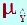2.  The  predicted value of y for an individual at a particular value of  x.

This is written  as Ŷ

It is still the same number just different symbols!!

This information is given in the data  window and not the output window.

 pre_1 sep_1 lmci_1 umci_1 lici_1 uici_1 Predicted  value standard  errorof thepredicted value lower limit confidence interval m = mean upper limit confidence interval m = mean lower limit prediction interval i = individual upper limit prediction interval i =  individual

Back to questions

(f) What is the 95%  confidence interval for the mean value of  Ŷ   when x =  ?

The confidence interval  for the population mean of all y values associated with the value of x  chosen.

It is similar to all other  confidence intervals in its interpretation and it involves a t-value and  standard error (sample standard error of predicted value).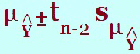This confidence interval  is found under the headings “lmci_1” and “umci_1” in the data window. This would  give the upper and lower limits of the confidence interval so in an examination,  it will probably be a different level of confidence i.e. 90% rather than the  output’s 95%. This means that you will look up the 90% t-value in tables. All  the other values remain the same so can be found in the output.

This value,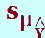is found in the output under the heading “sep_1”
“sep_1” is the sample standard error of the predicted value.

The value,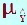is found in the output  under the heading “pre_1”
“pre_1”  is the predicted value.

The “_1” is just in case  you have more than one confidence or prediction interval so they can be  distinguished from each other.

You may be given the value  ofin the output,  you can findby the regression line or under the heading  “pre_1” and then substitute the values into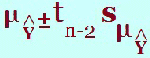along with the  t-value.

Back to questions

(g) What is the 95%  prediction interval for   Ŷ  when x =  ?

The prediction interval is  an interval of a prediction for an individual for a certain value of x.  This is  more variable than the confidence interval as we are dealing with individuals  rather than averages. Here is the formula and you can see the extra term   ‘s2

•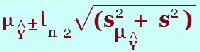where

The “ s ” is  standing for the sample standard error of the estimate

The  “is standing for the sample  standard error of the predicted  value

This prediction interval  is found under the headings “lici_1” and  “uici_1”  in the data window.  This would  give the upper and lower limits of the prediction interval so in an examination,  it will probably be a different level of prediction i.e. 90%  rather than the default output 95%. Once again look up the 90%  t-value and substitute in with all the  other values from the output.

In the output, the symbol  “i” is used to denote individual.

The value,is found in the output  under the heading “sep_1”
“sep_1” is the sample Standard error of the predicted value.

The value,  Ŷ  is found in the output under the heading ”pre_1”
“pre_1” is the  predicted value.

The value, s, is found in  the table below:

Model

# R  Square

Square

Std Error of
the Estimate

Durbin-  Watson

1

.941

.886

.867

.32121

2.321

Once again you will just  have to substitute the values into the equation

•The values of  Ŷ  andare different ways of expressing the predicted  value.

I hope you followed this  quick explanation of the SPSS output for regression.

Back to questions[Home]